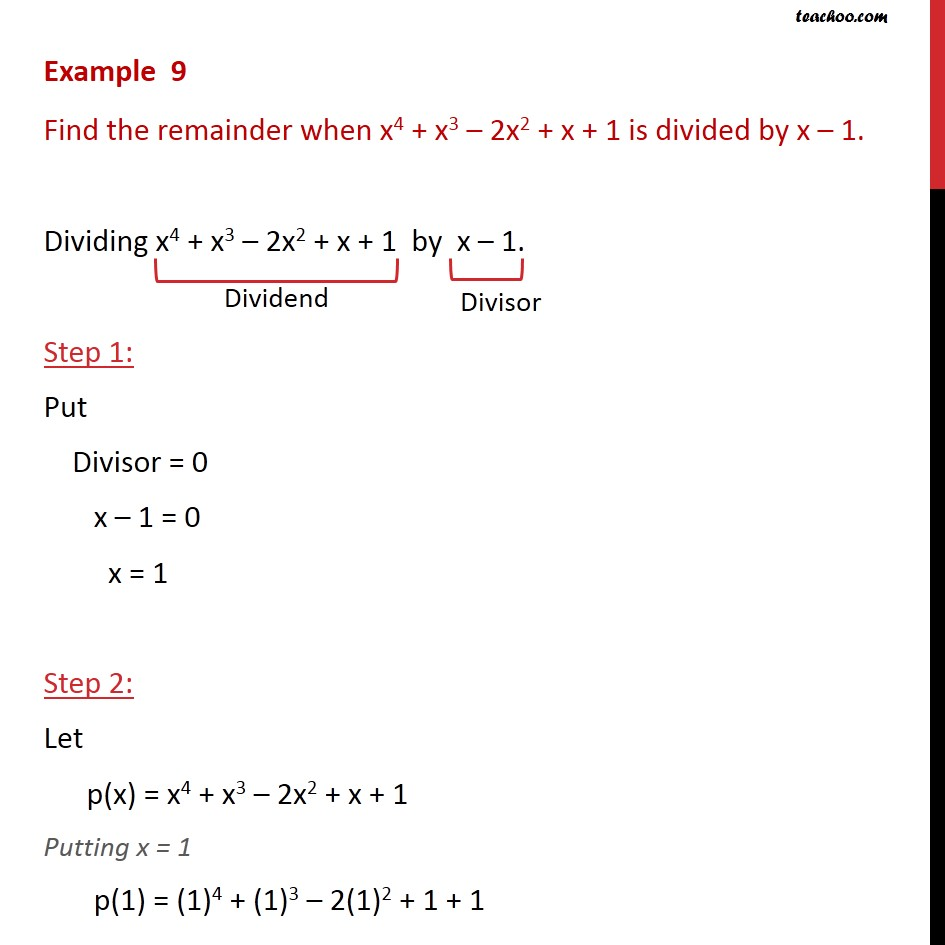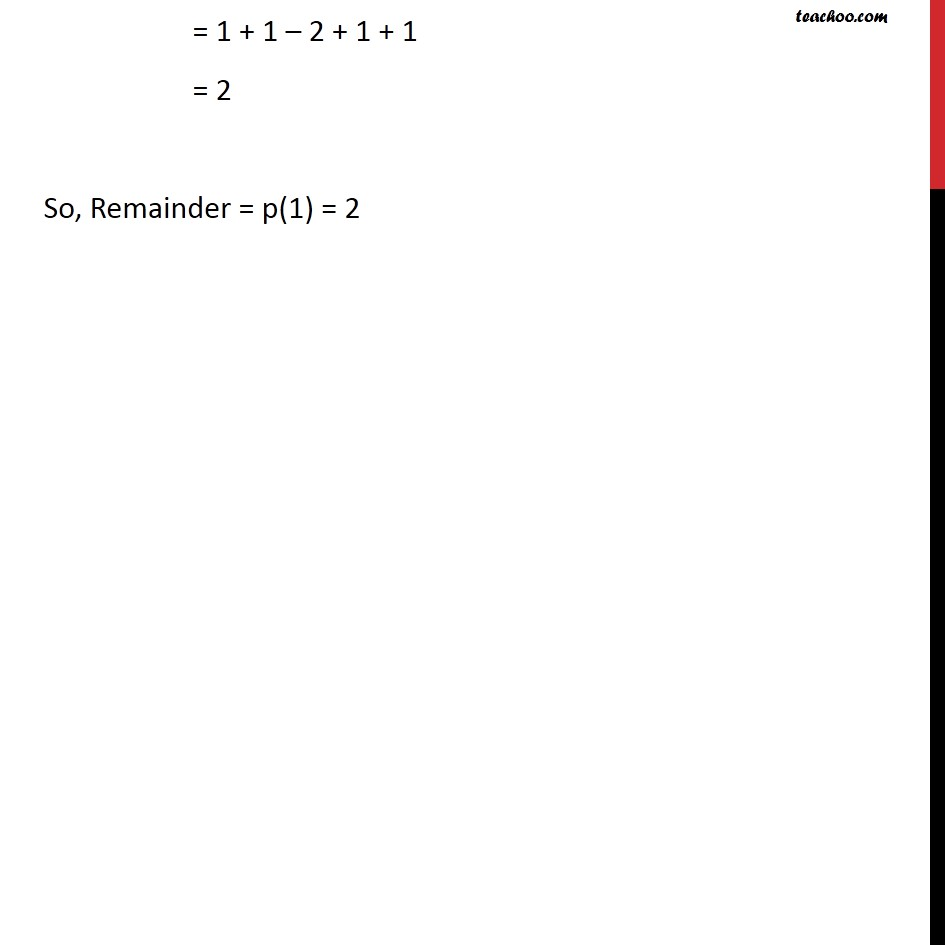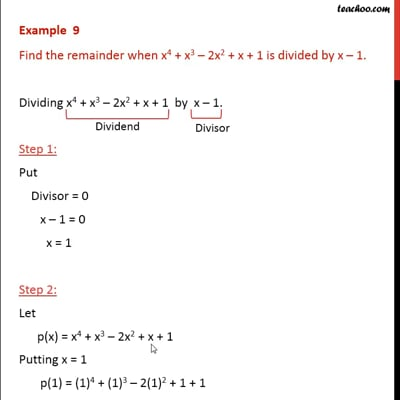Examples

Chapter 2 Class 9 Polynomials
Serial order wiseThis video is only available for Teachoo black users

Solve all your doubts with Teachoo Black (new monthly pack available now!)

### Transcript

Example 9 Find the remainder when x4 + x3 2x2 + x + 1 is divided by x 1. Dividing x4 + x3 2x2 + x + 1 by x 1. Step 1: Put Divisor = 0 x 1 = 0 x = 1 Step 2: Let p(x) = x4 + x3 2x2 + x + 1 Putting x = 1 p(1) = (1)4 + (1)3 2(1)2 + 1 + 1 = 1 + 1 2 + 1 + 1 = 2 So, Remainder = p(1) = 2